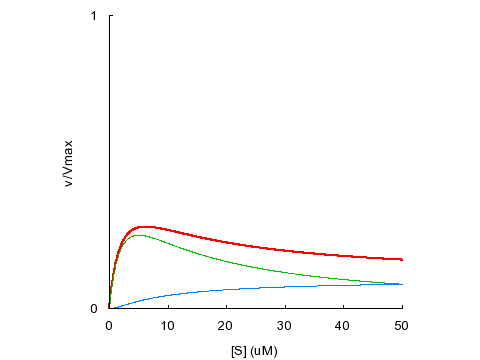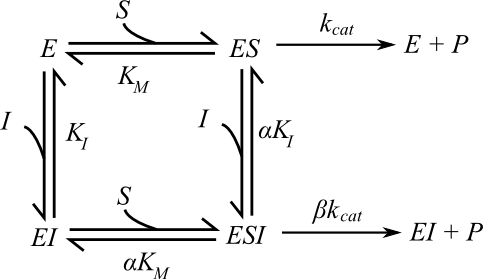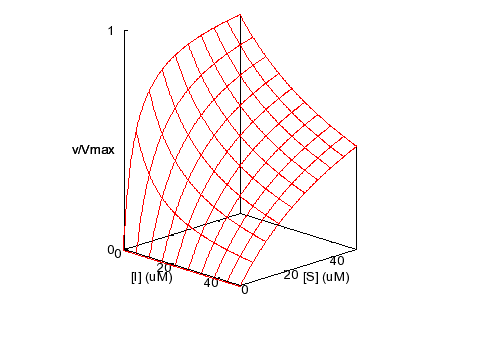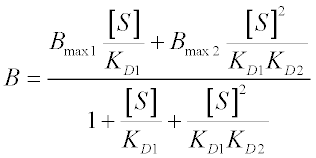# Michaelis-Menten Kinetics and Briggs-Haldane Kinetics

## Introduction

The Michaelis-Menten model (1) is the one of the simplest and best-known approaches to enzyme kinetics. It takes the form of an equation relating reaction velocity to substrate concentration for a system where a substrate S binds reversibly to an enzyme E to form an enzyme-substrate complex ES, which then reacts irreversibly to generate a product P and to regenerate the free enzyme E. This system can be represented schematically as follows:

$$E + S \rightleftharpoons ES \rightarrow E + P$$

The Michaelis-Menten equation for this system is:

$$v = \dfrac{V_{max}\;[S]}{K_M + [S]}$$

Here, Vmax represents the maximum velocity achieved by the system, at maximum (saturating) substrate concentrations. KM (the Michaelis constant; sometimes represented as KS instead) is the substrate concentration at which the reaction velocity is 50% of the Vmax. [S] is the concentration of the substrate S.

This is a plot of the Michaelis-Menten equation’s predicted reaction velocity as a function of substrate concentration, with the significance of the kinetic parameters Vmax and KM graphically depicted.Figure 1: Michaelis-Menten model: enzyme kinetic reaction velocity

The best derivation of the Michaelis-Menten equation was provided by George Briggs and J.B.S. Haldane in 1925 (2), and a version of it follows:For the scheme previously described, kon is the bimolecular association rate constant of enzyme-substrate binding; koff is the unimolecular rate constant of the ES complex dissociating to regenerate free enzyme and substrate; and kcat is the unimolecular rate constant of the ES complex dissociating to give free enzyme and product P. Note that kon has units of concentration-1time-1, and koff and kcathave units of time-1. Also, by definition the dissociation binding constant of the ES complex, KD is given by koff/kon (and so has units of concentration). Once these rate constants have been defined, we can write equations for the rates of change of all the chemical species in the system:

$$\dfrac{d[S]}{dt} = -k_{on} [E] [S] + k_{off} [ES]$$

$$\dfrac{d[E]}{dt} = -k_{on} [E] [S] + (k_{off} + k_{cat}) [ES]$$

$$\dfrac{d[P]}{dt} = k_{cat} [ES]$$

$$\dfrac{d[ES]}{dt} = k_{on} [E] [S] - (k_{off} + k_{cat}) [ES]$$

The last of these equations – describing the rate of change of the ES complex – is the most important for our purposes. In most systems, the ES concentration will rapidly approach a steady-state – that is, after an initial burst phase, its concentration will not change appreciably until a significant amount of substrate has been consumed. This steady-state approximation is the first important assumption involved in Briggs and Haldane’s derivation. This is also the reason that well-designed experiments measure reaction velocity only in regimes where product formation is linear with time. As long as we limit ourselves to studying initial reaction velocities, we can assume that [ES] is constant:

$$\dfrac{d[ES]}{dt} = 0$$

$$\Longrightarrow\ k_{on} [E][S] = (k_{off} + k_{cat}) [E][S]$$

In order to determine the rate of product formation (d[P]/dt = kcat[ES]), we need to rearrange the equation above to calculate [ES]. We know that the free enzyme concentration [E] is equal to the total enzyme concentration [ET] minus [ES]. Making these substitutions gives us:

$$k_{on} ([E_T] - [ES]) [S] = k_{off} + k_{cat} [ES]$$

$$k_{on} [E_T] [S] - k_{on} [ES] [S] = k_{off} + k_{cat} [ES]$$

$$k_{on} [E_T] [S] = k_{off} + k_{cat} [ES] + k_{on} [ES] [S]$$

$$[ES] = \dfrac{k_{on} [E_T] [S]}{(k_{off} + k_{cat}) + k_{on} [S]} = \dfrac{[E_T] [S]}{\left(\dfrac{k_{off} + k_{cat}}{k_{on}\right) + [S]}}$$

$$\Longrightarrow\ v = k_{cat} [ES] = \dfrac{k_{cat} [E_T] [S]}{\left(\dfrac{k_{off} + k_{cat}}{k_{on}\right) + [S]}}$$

We now make a couple of substitutions to arrive at the familiar form of the Michaelis-Menten equation. Since Vmax is the reaction velocity at saturating substrate concentration, it is equal to kcat [ES] when [ES] = [ET]. We also define KM in terms of the rate constants as follows:

$$V_{max} = k_{cat} \; [E_T]; \; K_M = \dfrac{k_{off} + k_{cat}}{k_{on}}$$

$$v = \dfrac{V_{max} [S]}{K_M + [S]}$$

Note that [S] here represents the free substrate concentration, but typically is assumed to be close to the total substrate concentration present in the system. This second assumption is the free ligand approximation, and is valid as long the total enzyme concentration is well below the KM of the system. If this condition is not met (for instance, with a very high-affinity substrate), then the quadratic (or ‘Morrison’) equation must be used instead.

Comparing KM [= (koff + kcat)/kon] and KD [= koff/kon], it is obvious that KM must always be greater than KD. Michaelis and Menten assumed that substrate binding and dissociation occurred much more rapidly than product formation (kcat << koff, the rapid equilibrium approximation), and that therefore the KM would be very close to the KD. The larger the kcat is relative to koff, the greater the difference between KD and KM. Briggs and Haldane made no assumptions about the relative values of koff and kcat, and so Michaelis-Menten kinetics are a special case of Briggs-Haldane kinetics. The opposite extreme, where kcat >> koff, is called Van Slyke-Cullen behavior (3).

## Quadratic Velocity Equation for Tight-Binding Substrates

Three assumptions are implicit in Michaelis-Menten kinetics: the steady-state approximation, the free ligand approximation and the rapid equilibrium approximation. (The Briggs-Haldane approach frees us from the last of these three.)

$$v = \dfrac{V_{max} [S]}{K_M + [S]}$$

Now consider the second assumption in the list: the free ligand approximation. Remember that this approximation states that the free substrate concentration (the [S] in the Michaelis-Menten equation) is close to the total substrate concentration in the system – which is, in fact, the true independent variable in most experimental set-ups. After all, we know how much substrate we’ve added to reaction mixture, but we don’t know a priori how much of that substrate remains free in solution at steady-state. The free ligand approximation does not hold when a substrate’s KM is lower than the total enzyme concentration, because at low substrate concentrations, a significant fraction of substrate will be bound to the enzyme.For such unusual cases, we derive a kinetic equation from the scheme above without resorting to the free ligand approximation. The rates of change of the various species are given by four differential equations:

$$\dfrac{d [S]}{dt} = -k_{on} [E][S] + k_{off} [ES]$$

$$\dfrac{d [E]}{dt} = -k_{on} [E][S] + \left(k_{off} +k_{cat}\right)[ES]$$

$$\dfrac{d [P]}{dt} = k_{cat} [ES]$$

$$\dfrac{d [ES]}{dt} = k_{on} [E][S] - \left(k_{off} +k_{cat}\right)[ES]$$

We can assume that ES levels achieve steady-state:

$$\dfrac{d [ES]}{dt} = 0\; \Longrightarrow\; k_{on} [E][S] =\left(k_{off} +k_{cat}\right)[ES]$$

Given that free enzyme concentration [E] equals total enzyme concentration [ET] minus [ES] and that [S] equals total enzyme concentration, we have:

$$k_{on} \left([E_T] - [ES]\right)\left([S_T] - [ES]\right) = \left(k_{off} + k_{cat}\right)[ES]$$

A little algebra gives us:

$$\left([E_T] - [ES]\right)\left([S_T] - [ES]\right) = \dfrac{k_{off} + k_{cat}}{k_{on}} [ES] = K_M [ES]$$

$$[E_T][ES] - \left([E_T] + [S_T]\right) [ES] + [ES]^{2} = K_M [ES]$$

$$[ES]^{2} - \left([E_T] + [S_T] +[K_M]\right) [ES] + [E_T][S_T] = 0$$

This is a quadratic equation of the form a2x + bx + c = 0, whose roots are given by: $$\;\dfrac{\left(-b \pm \sqrt{b^2 - 4ac}\right)}{2a}$$

Making the following substitutions and choosing the relevant (saturable) root gives us:

$$a = 1$$

$$b = - \left([E_T] + [S_T] + K_M \right)$$

$$c = [E_T][S_T]$$

$$[ES] = \dfrac{\left([E_T] + [S_T] + K_M \right) - \sqrt{\left([E_T] + [S_T] + K_M \right)^2 - 4[E_T][S_T]}}{2}$$

$$v = k_{cat} [ES] = \dfrac{V_{max}}{[E_T} [ES]$$

$$v = V_{max} \dfrac{\left([E_T] + [S_T] + K_M \right) - \sqrt{\left([E_T] + [S_T] + K_M \right)^2 - 4[E_T][S_T]}}{2 [E_T]}$$

This is the quadratic velocity equation, sometimes also called the tight-binding equation or the Morrison equation (4). As the KM becomes larger than [ET], the curve described by the quadratic equation approaches the hyperbola described by the Michaelis-Menten equation. As the KM becomes lower, the inflection point of the curve (located where [ST] = [ET]) becomes progressively sharper. This is illustrated in the plot below, where the KM varies from 5 times the total enzyme concentration ([ET] = 1 μM) to one-hundredth of [ET]. Differences in affinity between very tight-binding substrates are reflected in the sharpness of the inflection, meaning that KM values recovered from fits to experimental data are disproportionately sensitive to error in the data points surrounding the inflection point. As a rule of thumb, the quadratic equation should be used in preference to the Michaelis-Menten equation whenever the KM is less than five-fold larger than [ET].

Derivations of this type that do not use the free ligand approximation grow rapidly more complicated for more complex kinetic systems. For anything more complicated than a one-site model, it is better to use kinetic simulations as detailed in part II of this guide.

Building on the derivation of Michaelis-Menten kinetics, we now turn to enzymes with multiple substrate-binding sites.

## Multiple Binding: Sequential Models

Early work in this regard was carried out by Adair and Pauling, operating under the rapid equilibrium approximation. Remember that this assumption states that all substrate binding and dissociation steps happen much more rapidly than catalytically productive steps. In 1954, King and Altman showed how to solve any kinetic system without resorting to this approximation, but this is unnecessarily complicated for our purposes. Even if the rapid equilibrium approximation does not hold for our system, the forms of the equations we derive will not change – instead, the significance of the various KM values will be different. This is analogous to the difference between KM (or KD) in the Michaelis-Menten model and KM in the Briggs-Haldane model.

We begin with the simplest model of multiple binding: a two-site sequential model. Here, an enzyme E can bind a single molecule of substrate S to form a singly-occupied complex ES with equilibrium dissociation constant KD1. ES can either react irreversibly to form product P with rate kcat1, or can bind a second substrate molecule S to form a doubly-occupied complex ESS with dissociation constant KD2. In turn, ESS can irreversibly form P with rate kcat2. This is represented schematically below:In order to calculate the reaction velocity for this system, we need to know the relative concentrations of the active species ES and ESS. We will describe a straightforward derivation of these quantities, and then a shortcut that allows one to quickly solve more complicated kinetic systems.

From the definitions of the two dissociation constants, we have:

$$K_{D_1} = \dfrac{[E][S]}{[ES]} \Longrightarrow [ES] = \dfrac{[E][S]}{K_{D_1}}$$

$$K_{D_2} = \dfrac{[E][S]}{[ESS]} \Longrightarrow [ESS] = \dfrac{[ES][S]}{K_{D_2}} = \dfrac{[E][S]^2}{K_{D_1}K_{D_2}}$$

Relative to the total enzyme concentration, ET:

$$\dfrac{[ES]}{[E_T]} =\dfrac{[ES]}{[E] + [ES] + [ESS]} = \dfrac{\dfrac{[E][S]}{K_{D_1}}}{[E] + \dfrac{[E][S]}{K_{D_1}} + \dfrac{[E][S]^2}{K_{D_1} K_{D_2}}} = \dfrac{\dfrac{[S]}{K_{D_1}}}{1 + \dfrac{[S]}{K_{D_1}} + \dfrac{[S]^2}{K_{D_1} K_{D_2}}}$$

$$\dfrac{[ESS]}{[E_T]} = \dfrac{[ESS]}{[E] + [ES] + [ESS]} = \dfrac{\dfrac{[E][S]^2}{K_{D_1} K_{D_2}}}{[E] + \dfrac{[E][S]}{K_{D_1}} + \dfrac{[E][S]^2}{K_{D_1} K_{D_2}}} = \dfrac{\dfrac{[S]^2}{K_{D_1} K_{D_2}}}{1 + \dfrac{[S]}{K_{D_1}} + \dfrac{[S]^2}{K_{D_1} K_{D_2}}}$$

The total reaction velocity for this system is given by (where Vmax1 = kcat1[ET] and Vmax2 = kcat2[ET]):

$$\nu = \dfrac{V_{max_1} \dfrac{[S]}{K_{D_1}} + V_{max_2} \dfrac{[S]^2}{K_{D_1} K_{D_2}}}{1 + \dfrac{[S]}{K_{D_1}} + \dfrac{[S]^2}{K_{D_1} K_{D_2}}}$$

This graph shows the relative concentrations of the two active species as a function of substrate concentration, when both KDs are set to 10 μM. Note the initial increase and subsequent decrease in the level of ES, while the concentration curve for ESS is sigmoidal. Therefore, if Vmax1 (for ES) is much greater than Vmax2 (for ESS) then the system will exhibit substrate inhibition kinetics; if Vmax2 is much greater than Vmax1 then the system will exhibit sigmoidal kinetics. We will return to this idea when we discuss allosterism in more detail.Figure 3: KDs = 10μM

There is a quicker way to calculate reaction velocity equations of this type. To return to the scheme of a two-site sequential model:Each species in the system has a relative concentration term that appears in the denominator of the velocity equation, while terms from all active species appear in the numerator. To find the relative concentration term of any species, simply work your way backwards from that species to the free enzyme; the specific term is equal to the product of all the substrate molecules that bind, divided by all the equilibrium constants in the chain. So the specific term for ES is [S]/KD1 and the term for ESS is [S]2/KD1KD2. The specific term for free enzyme is always 1.

The denominator of the velocity equation is the sum of all specific terms: 1 + [S]/KD1 + [S]2/KD1KD2; the numerator is the sum of specific terms for catalytically active species, each weighted by their respective Vmax values – and voila:

$$\nu = \dfrac{V_{max_1} \dfrac{[S]}{K_{D_1}} + V_{max_2} \dfrac{[S]^2}{K_{D_1} K_{D_2}}}{1 + \dfrac{[S]}{K_{D_1}} + \dfrac{[S]^2}{K_{D_1} K_{D_2}}}$$

## Multiple Binding: Random-ordered Models

In a two-distinct-site random-ordered model, there are two substrate binding sites on the enzyme, each with their own KDs. Once either site is occupied, the substrate can bind to the other site with an altered KD. If the substrate binding at one site enhances the affinity of binding at another site, then the enzyme exhibits positive cooperativity. If the reverse is true (binding at one site decreases the affinity of the other site), the enzyme shows negative cooperativity.

Continuing from our discussion of Sequential Binding Models, we move to a slightly more rigorous description of multiple binding: random-ordered models.In the scheme above, ES and SE are the two singly-occupied complexes and SES is the doubly-occupied complex. ES and SES are both productive: the parameter β reflects how productive the SES complex is relative to ES, in that values below 1 imply substrate inhibition and values above 1 imply activation. The cooperativity parameter α shows how binding at one site affects the affinity at the other: values below 1 indicate increased affinity (positive cooperativity) and values above 1 indicate negative cooperativity.

Extending the quick derivation at the end of the previous section on sequential binding, the specific terms for ES, SE and SES are [S]/KD1,[S]/KD2, and[S]2/αKD1KD2 respectively. Therefore, the velocity equation for the system is:If one binding site is much higher-affinity than the other, the specific term associated with the weaker-binding (higher KD) site is negligible and can therefore be omitted from this equation, which then resembles the velocity equation previously derived for the two-site sequential model. In other words, sequential binding models are a special case of random-ordered models where one binding site is much higher-affinity than the other (KD1 >> KD2 or KD1 << KD2).

Although sequential models are less general than random-ordered models, they have fewer free parameters and so are easier to use when fitting experimental data. This means that, in cases where they are applicable, sequential models are often a better choice than random-ordered models when it comes to analyzing experimental data.

## Homotropic Allosterism

Here, we use the random-ordered binding model to describe how common forms of atypical (non-hyperbolic) allosteric behavior may arise. We focus here on homotropic allosterism, where multiple molecules of a singly chemical species can bind the enzyme.We know that the scheme above, showing a two-site random-ordered model, is described by the following velocity equation:Different combinations of the parameters α and β can produce hyperbolic or allosteric behavior. For instance, if we set KD1 = KD2 = 5 μM with α = 1 (no cooperativity in binding) and β = 1 (ES and SES have identical activity), the overall velocity equation corresponds to a hyperbola with an apparent KM of 5 μM.Figure 4: KD1 = KD2 = 5 μM, α = 1, β = 1

Total velocity is shown in red, with contributions from ES in green and SES in blue. In the absence of binding cooperativity and substrate activation, with equal KDs for both binding sites, the velocity curve appears hyperbolic.

Sigmoidal kinetics can be caused by positive binding cooperativity (α < 1) or by substrate activation (β > 1).Figure 5: KD1 = KD2 = 5 μM, α = 0.1, β = 1

Sigmoidal kinetics can result from positive binding cooperativity.Figure 6: KD1 = KD2 = 5 μM, α = 1, β = 5

Sigmoidal kinetics can also result from substrate activation, even without binding cooperativity.

Biphasic kinetics can result from negative binding cooperativity (α > 1):Figure 7: KD1 = KD2 = 5 μM, α = 10, β = 1

Substrate inhibition occurs when SES is less active than ES (β < 1):Figure 8: KD1 = KD2 = 5 μM, α = 1, β = 0.1

## Inhibition and Allosterism

Random-ordered models can easily be adapted to describe many common modes of enzyme inhibition and activation by chemical species different from the substrate. The following scheme is a generalized model of inhibition that can describe competitive, uncompetitive, mixed and non-competitive inhibition, as well as heterotropic activation.The specific terms for ES, EI and ESI are [S]/KM,[I]/KI, and[S][I]/αKMKI respectively. This leads to the following velocity equation for the general case:This equation represents a 3-D surface with [S] and [I] as independent variables.

The parameter β describes the extent of inhibition (when β < 1) or the extent of activation (when β > 1). This system approaches competitive inhibition (where inhibitor binds to the free enzyme but not the ES complex) when α >> 1 and so KI << αKI. Uncompetitive inhibition, where I binds ES but not E, is achieved when KI >> αKI. Noncompetitive inhibition occurs when α = 1, and I binds E and ES with equal affinities. All other combinations of parameters represent mixed inhibition.Figure 9: Competitive Inhibition: KM = 5 μM, KI = 5 μM, α = 1000, β = 0Figure 10: Uncompetitive Inhibition: KM = 5 μM, KI = 5000 μM, α = 0.001, β = 0Figure 11: Non-competitive Inhibition: KM = 5 μM, KI = 5 μM, α = 1, β = 0Figure 12: Partial Mixed Inhibition KM = 5 μM, KI = 5 μM, α = 5, β = 0.2Figure 13: Activation KM = 5 μM, KI = 5 μM, α = 0.2, β = 2.5

More complex models that incorporate multiple binding sites for substrates and inhibitors can be constructed using the techniques outlined here, but this is left as an exercise for the reader.

## Translating Between Velocity Equations and Binding Equations

So far we have devoted all of our attention to modeling turnover kinetics. Of course, many experiments focus not on catalysis but on equilibrium binding, using absorbance or fluorescence changes induced by substrate binding as observable signals. The strategies we used to derive velocity equations for kinetic models are easily adapted to derive binding equations for equilibrium models. Basically, all KMs are replaced by KDs and all Vmax values are replaced by appropriate scaling factors for the observed signals.

For example, consider a two-site sequential equilibrium binding model:If the ES and ESS species have differential responses to the experimental technique used, they will each have a maximal response Bmax1 and Bmax2. Then the equilibrium binding equation (where B is the observed signal) is:This translation can be done for any of the kinetic models we have covered previously.

1. Michaelis, L.,and Menten, M. (1913) Die kinetik der invertinwirkung, Biochemistry Zeitung 49, 333-369.
2. Briggs, G. E., and Haldane, J. B. (1925) A Note on the Kinetics of Enzyme Action, Biochem J 19, 338-339.
3. Van Slyke, D. D., and Cullen, G. E. (1914) The Mode of Action of Urease and of Enzymes in General, J Biol Chem 19, 141-180.
4. Morrison, J. F. (1969) Kinetics of the reversible inhibition of enzyme-catalysed reactions by tight-binding inhibitors, Biochimica et Biophysica Acta (BBA) - Enzymology 185, 269-286.

## Contributors

• Abhinav Nath (Atkins group) University of Washington